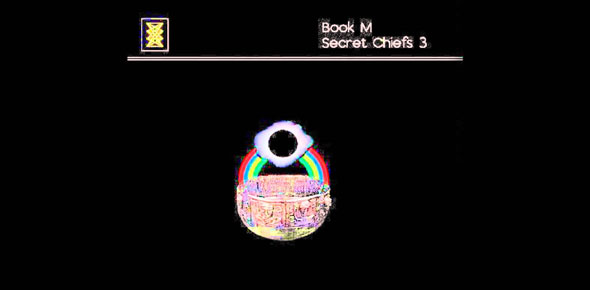# Book M Ch 4 Formula Quiz

7 Questions | Total Attempts: 176SettingsThis is a pop quiz over the formulas we've been using in this chapter. All answers will be in the nearest WHOLE number , not tenths. Put ONLY the numbers in, not the units (such as Joules or Watts).

• 1.
How much power is needed to cut a lawn in 50 minutes if the work involved is 100,000J? Hint: you must convert minutes to seconds. 50 minutes times 60 seconds ? ___Watts (put only the numeric value to the nearest WHOLE number).
• 2.
As you push a lawn mover, the horizontal force is 300 N. If you push the mower a distance of 500 M, how much work do you do?  _____ J
• 3.
A librarian lifts a box of books that weighs 93 N a distance of 1.5 m. How much work does she do? ____J
• 4.
A teacher does 140 J of work in 20 s. How much power did he use?  ____W
• 5.
You do 100 J of work in pulling out a nail with a claw hammer. If the hammer does 70 J of work, what is the hammer's efficiency? ______%
• 6.
Find the efficiency of a machine if the input work is 150 J and the output work is 90 J.  _____%
• 7.
To lift a crate, a pulley system exerts a force of 2,750 N. Find the mechanical advantage of the pulley system if the input force is 250 N.  ____
Related TopicsBack to top